# Cumulative SUM for Top Ten ItemsHi There,

I was wondering if you can point me in the right direction on finding how to do the below 😃

I am trying to build a report that can show me just the Vendor that make the 70% of the Total Expense. I have 3 dimensions (VEndor LIST, Cost Center and Product). As a Line items I have the "Expense" and "% of Expense". From this data I want to show on a Dashboard just the Vendors that make the 70% of the expense, I have tried cumulative but it doesn't work or maybe I just did it wrong.....Thank you!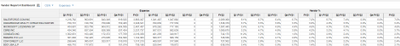Tagged:

•Great start but lets work on getting you to the final solution.

So with the rankings in place you now want to sum up the top performers until the cumulative % exceeds 70%?

Therefore, we will combine an IF THEN ELSE function with RANKCUMULATE.

We start with the IF THEN ELSE so that we can direct the outcome to either continue with the sum or to exist and return a zero if the outcome meets the query conditions.

Next we need to consider the query that will direct the function to the TRUE or FALSE outcome and place the most probable outcome as true and least probable as false. This approach will maximise the efficiency of the formula as the calculation will be able to exist each iteration at the earliest point and move onto the next one, therefore speeding it up.

Next is then understanding how we use RANKCUMULATE to sum the % in order of the ranking that you have already produced. This will form part of the query conditions and also one of the outcomes.

Putting it all together we should have something like the following;

=IF RANKCUMULATE(Vendor %, Rank, ASCENDING)>0.8 THEN 0 ELSE RANKCUMULATE(Vendor %, Rank, ASCENDING, TRUE, Group By)

I would use the keyword ASCENDING as you are summing up from rank 1 onwards with rank 1 being the largest Vendor %.

The above will continue to generate the FALSE outcome of  RANKCUMULATE(Vendor %, Rank, ASCENDING, TRUE, Group By) upto the point where the RANKCUMULATE generates a sum greater than 80%.

I have placed the RANKCUMULATE as the false outcome as the majority of outcomes will be FALSE.

If this is not true then swap the inequality to be > and swap the outcomes over.

Let me know how you get on.

•You will need to combine SORT the vendors and RANK them to place your vendors into descending order.

Finally use RANKCUMULATE to SUM in order of descending rank the vendors which contribute the most.

Use IF THEN ELSE to stop the SUM when value exceeds a threshold. In this case 70%.

These functions will all work across multiple dimensions.

•Hi Chris @ChrisAHeathcote

Thanks for the help. I did the RANK and it worked 😃 but it seems that is just doing it at a higher level....below on the Picture If I select Corporate as a Concenter then the RANK start at 8 but I think it is because when you put "All Cost Centers - Expenses" like the second picture you have RANK since 1 and so on...so it is being applied at a higher level.....Is that right?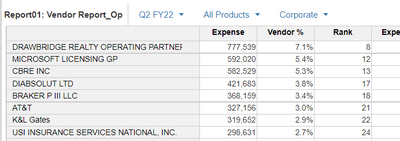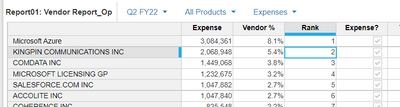Besides I am having issues with the "RANKCUMULATE", I was thinking that should be as follow RANCUMULATE("Vendor%", Expense, DESCENDING, ......) I am having issues with the threshold to stop the calcs up to 70%. Should I add one more line item to do the IF THEN ELSE Formula?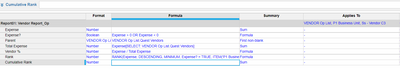Thanks for the help!

•I will let @ChrisAHeathcote finish this up, but one suggestion I would do, and it really doesn't mean a whole lot to the **** eye, but it will decrease the number of calculations the system has to do.  In your Expense? line item, change the formula to be Expense <> 0.  This way, you are not having to do multiple calculations (less than 0 OR greater than 0).  And OR's are always bad for performance.

Rob

•I went an apply a guidance from "Dynamic Ranking" Article and it helped me to "RANK" the vendor at the lowest Level for Product and Cost Center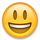. But now I don't know what to do on just having the RANKCUMULATE to SUM up to 80% of the Total Expenses. Thanks for the help.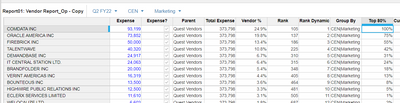A view of the "Blue Print" so far.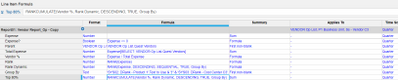Regards,

•Thank you @ChrisAHeathcote !!

It worked perfectly - I had no clue on how to use the IF THEN ELSE as I though that I needed to apply first SUM and then the formula with the RANCUMULATE (a little bit of misunderstanding on my end) .

I have just modify a little bit the formula =IF RANKCUMULATE(Vendor %, Rank, ASCENDING)>0.8 THEN 0 ELSE RANKCUMULATE(Vendor %, Rank, ASCENDING, TRUE, Group By) as it was given me 0% for the subgroups, so I added =IF RANKCUMULATE(Vendor %, Rank, ASCENDING, TRUE, Group By)>0.8 THEN 0 ELSE RANKCUMULATE(Vendor %, Rank, ASCENDING, TRUE, Group By)  and "Magic Happen".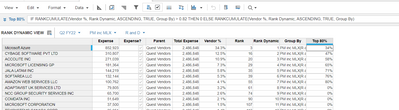Thanks! 😃

•Great. Glad that it worked out!